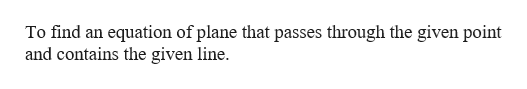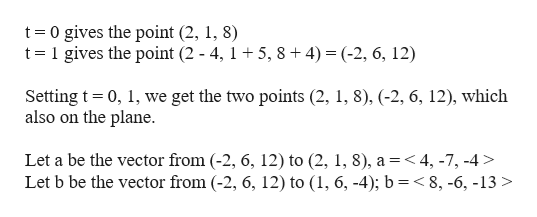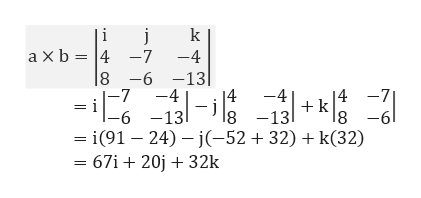# Find an equation of the plane.The plane that passes through (6, 0, –1) and contains the linex = 2 - 4t, y = 1 + 5t, z = 8 + 4t

Question
1 viewshelp_outlineImage TranscriptioncloseFind an equation of the plane. The plane that passes through (6, 0, –1) and contains the line x = 2 - 4t, y = 1 + 5t, z = 8 + 4t fullscreen
check_circle

Step 1

Given a point (6, 0, -1) and a line x = 2 - 4t, y = 1 + 5t, z = 8 + 4thelp_outlineImage TranscriptioncloseTo find an equation of plane that passes through the given point and contains the given line. fullscreen
Step 2

To begin with find 2 more points by setting the values for t,help_outlineImage Transcriptioncloset= 0 gives the point (2, 1, 8) t=1 gives the point (2 - 4, 1+ 5, 8+ 4) = (-2, 6, 12) Setting t= 0, 1, we get the two points (2, 1, 8), (-2, 6, 12), which also on the plane. Let a be the vector from (-2, 6, 12) to (2, 1, 8), a=<4, -7, -4 > Let b be the vector from (-2, 6, 12) to (1, 6, -4); b =< 8, -6, -13 > fullscreen
Step 3

The normal of the tw...help_outlineImage Transcriptionclose|i k a xb = 4 -7 -4 |8 -6 -13 -9- -7 -4 14 -4 14 -7 18 =i|-6 -13-8 -13 = i(91 – 24) – j(-52+32) + k(32) = 67i+ 20j + 32k -9- fullscreen

### Want to see the full answer?

See Solution

#### Want to see this answer and more?

Solutions are written by subject experts who are available 24/7. Questions are typically answered within 1 hour.*

See Solution
*Response times may vary by subject and question.
Tagged in
MathCalculus

### Other